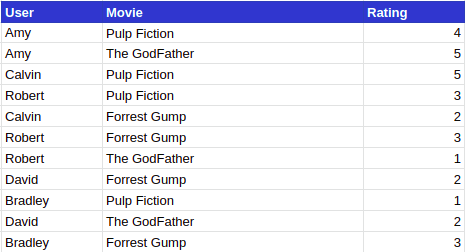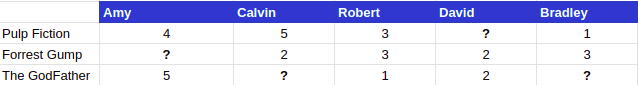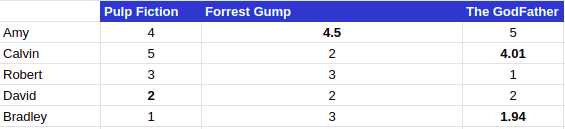{{announcement.body}}
{{announcement.title}}

# MachineX: Cosine Similarity for Item-Based Collaborative Filtering

DZone 's Guide to

# MachineX: Cosine Similarity for Item-Based Collaborative Filtering

### In this post, we will be looking at a method named Cosine Similarity for item-based collaborative filtering.

· AI Zone ·
Free Resource

Comment (4)

Save
{{ articles.views | formatCount}} Views

"A recommender system or a recommendation system (sometimes replacing "system" with a synonym such as a platform or an engine) is a subclass of information filtering system that seeks to predict the "rating" or "preference" a user would give to an item." — Wikipedia

In simple terms, a recommender system is where the system is capable of producing a list of recommendation with respect to an item. One of the ways to create a recommender system is through Collaborative Filtering, where the information is filtered by looking at the activity of other users. Most companies these days use recommender systems to provide better recommendations to the users.

Some of the examples are Amazon using a recommender system to provide a recommendation on the items or Netflix providing recommendations on next movies to watch after a user has seen a movie.

Collaborative Filtering is further divided into 2 parts:

1. User-Based Collaborative Filtering (UB-CF): Recommendations based on the calculating similarities of two users
2. Item-Based Collaborative Filtering (IB-CF): Recommendation based on calculating similarities of two items based on peoples rating of two items.

In this post, we will be looking at a method named Cosine Similarity for Item-Based Collaborative Filtering.

NOTE: Item-Based similarity doesn’t imply that the two things are like each other in case of attributes. Rather, it is similarity concerning how individuals treat the two given things in case of like or dislike.

## What Is Cosine Similarity?

Cosine similarity is a metric used to measure how similar the two items or documents are irrespective of their size. It measures the cosine of an angle between two vectors projected in multi-dimensional space. This allows us to measure the similarity of a document of any type. Due to a multi-dimensional array, any number of variables (which are treated as dimensions) can be used, which in turn supports large sized documents

Mathematically, the cosine of the angle of between two vectors is derived from the dot product of the two vectors divided by the product of the two vectors’ magnitude.

Since we are finding the cosine of two vectors the output will always range from -1 to 1, where -1 shows that two items are dissimilar and 1 shows that two items are completely similar. We will now see how we can use the Cosine Similarity measure to determine how similar the movies are.

## Why Cos(Θ)?

We can multiply two vectors only when they are in the same direction. So we make one “Point in the same direction” as the other by multiplying by Cos, which gives us the dot product of two vectors

``A.B = |a||b|Cos(Θ)``

### Example

Suppose we have movie ratings given by different users in a table format as shown below:Step 1: We create a sparse matrix where we write user-item ratings in a matrix formIn this matrix user, Amy has already rated and watched movies Pulp Fiction and The GodFather but hasn’t watched the movie, Forrest Gump. We will be using the above matrix for our example and will try to create an item-item similarity matrix using Cosine Similarity method to determine how similar the movies are to each other.

Step 2: To calculate the similarity between the movie Pulp Fiction (P) and Forrest Gump (F), we will first find all the users who have rated both the movies. In our case, Calvin (C), Robert (R) and Bradley (B) have rated the movies. We now create two vectors:

``````v1 =  5 C + 3 R + 1 B

v2 = 2 C + 3 R + 3 B``````

Therefore Cosine Similarity between movies Pulp Fiction and Forrest Gump is:

``cos(v1,v2) = (5*2 + 3*3 + 1*3) / sqrt[(25+9+1) * (4+9+9)] = 0.792``

Similarly, we can calculate the cosine similarity of all the movies and our final similarity matrix will be:

Step 3: Now we can predict and fill the ratings for a user for the items he hasn’t rated yet. So to calculate the rating of user Amy for the movie Forrest Gump, we will use the calculated similarity matrix along with the already rated movie by the user. Therefore, the rating would be:

``(4*0.792 + 5*0.8) / (0.792+ 0.8) = 4.5``

Hence, our final matrix would be:Hope, you enjoyed the post. Let me know your thoughts in the comments.

Topics:
ai, cosine similarity, ib-cf, item based collaborative filtering, machine learning, machine learning algorithm, machinex, tutorial, ub-cf

Comment (4)

Save
{{ articles.views | formatCount}} Views

Published at DZone with permission of Rahul Khanna . See the original article here.

Opinions expressed by DZone contributors are their own.